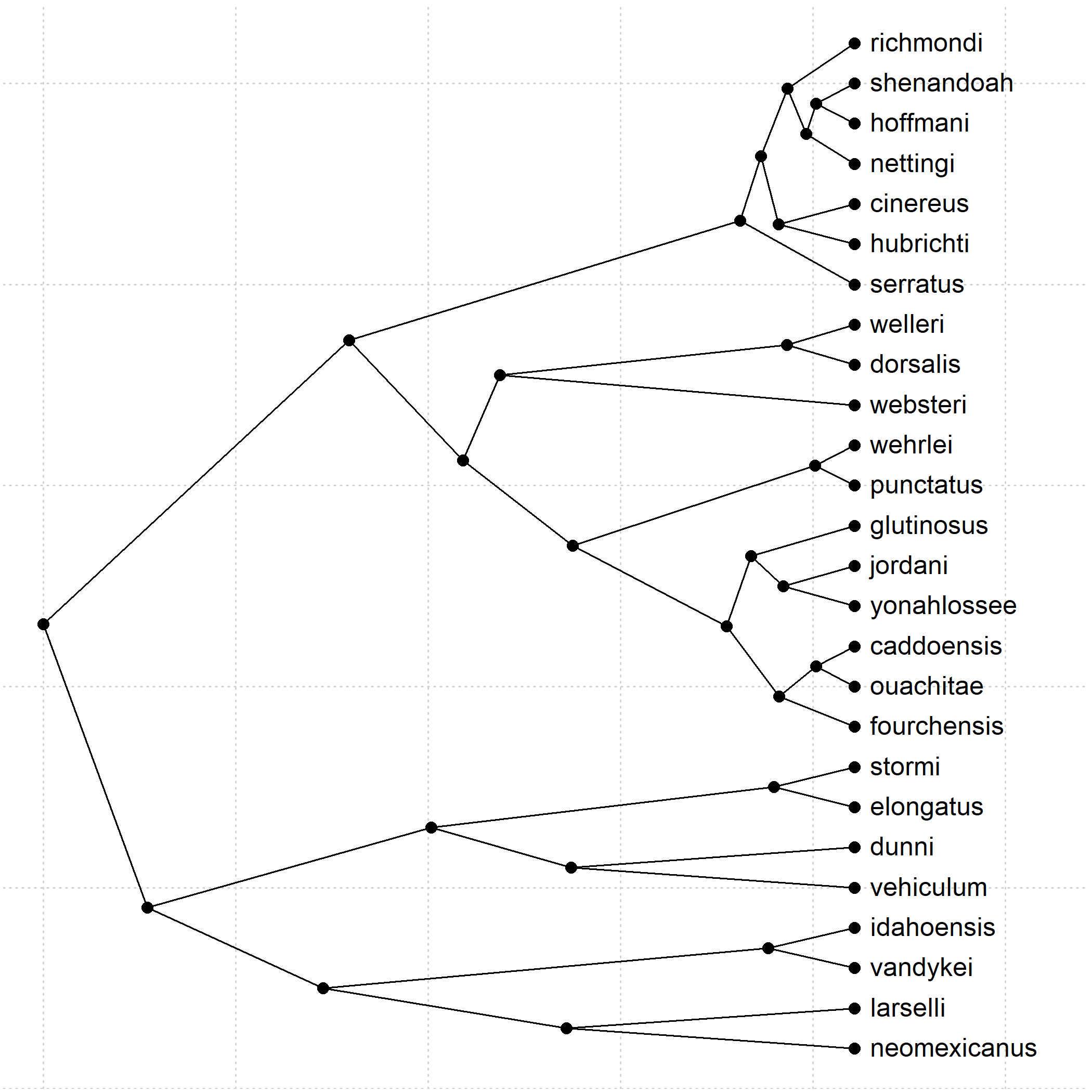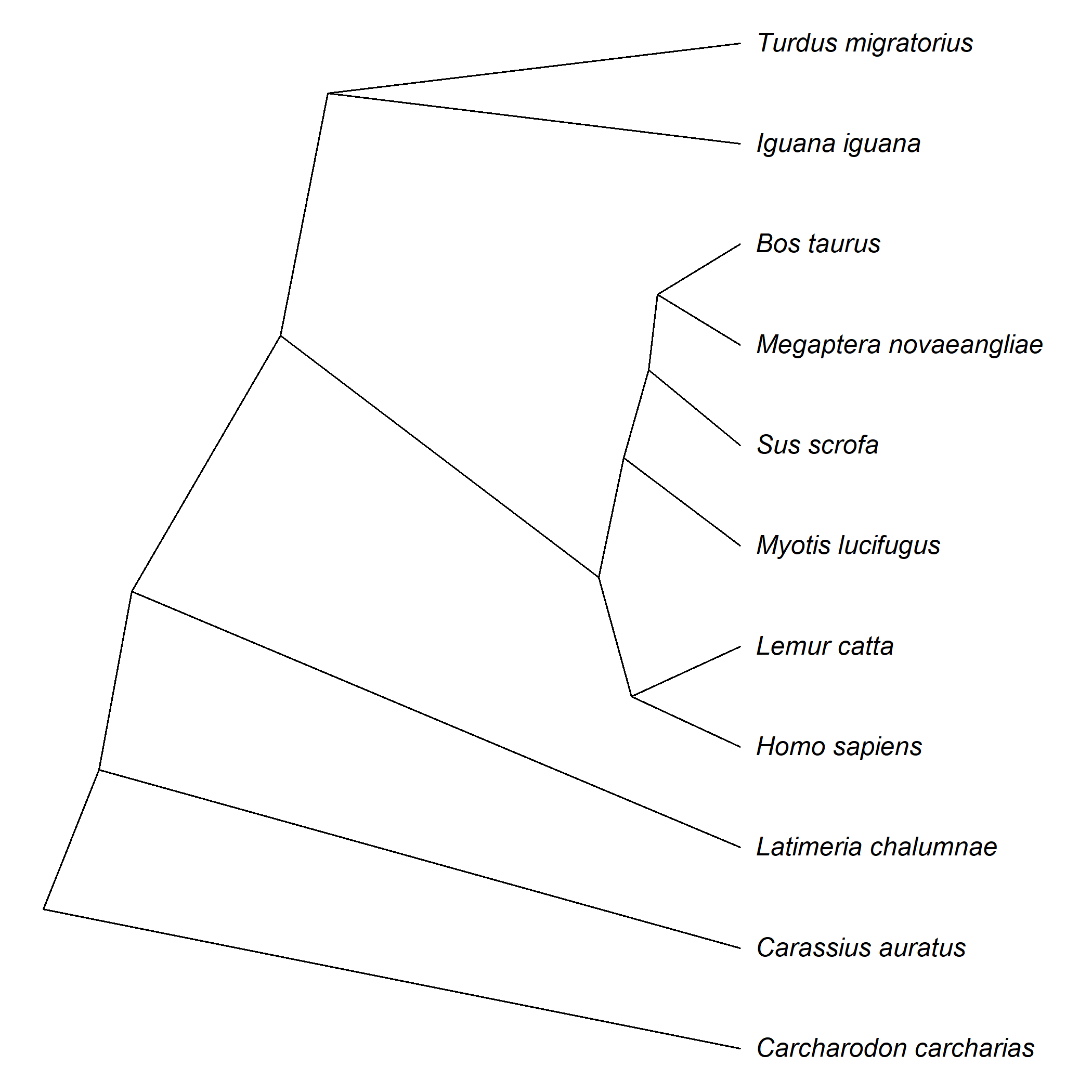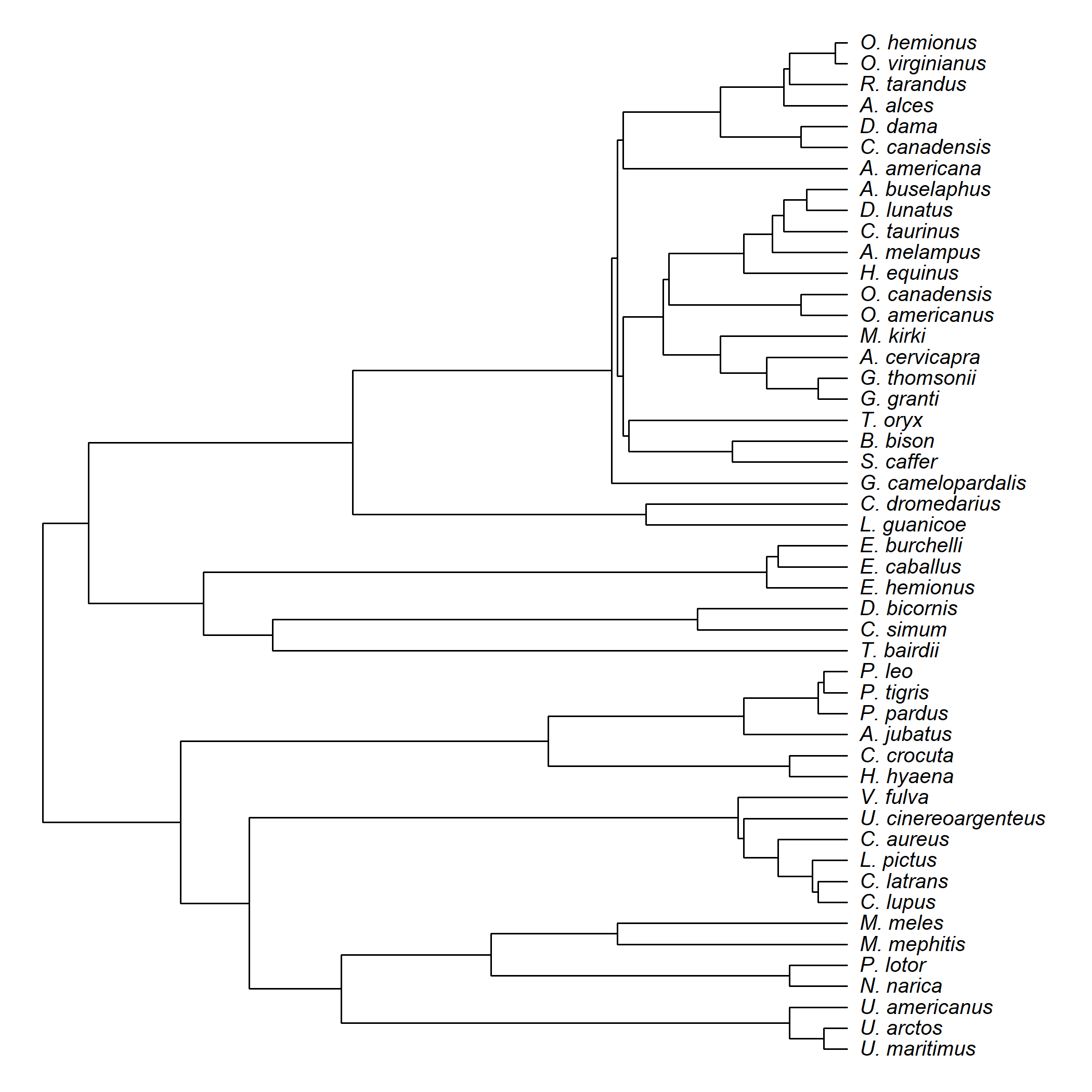## Wednesday, October 26, 2022

### Plotting a tree based on the coordinates computed internally by phytools or ape plotting functions

At the end of a recent phylogenetic comparative methods in R workshop at the University of Wyoming, I showed how to write a very simple function that uses internals of ape and phytools to plot a simplified tree.

I tweeted about it, and one of my followers asked me to post about it on my blog.

Today, a colleague asked me how we could go from this slanted tree to a square “phylogram-style” plot.

First, the algorithm.

``````## load phytools
library(phytools)
data(salamanders)
## call plotTree to compute the coordinates of our graph
plotTree(salamanders,plot=FALSE)
grid()
## pull the coordinates of our graph and plot them
pp<-get("last_plot.phylo",
envir=.PlotPhyloEnv)
## this is out "last_plot.phylo" object
str(pp)
``````
``````## List of 20
##  \$ type           : chr "phylogram"
##  \$ use.edge.length: logi TRUE
##  \$ node.pos       : num 1
##  \$ show.tip.label : logi TRUE
##  \$ show.node.label: logi FALSE
##  \$ font           : num 1
##  \$ cex            : num 1
##  \$ adj            : num 0
##  \$ srt            : num 0
##  \$ no.margin      : logi FALSE
##  \$ label.offset   : num 0.2
##  \$ x.lim          : num [1:2] 0 523
##  \$ y.lim          : num [1:2] 1 26
##  \$ direction      : chr "rightwards"
##  \$ tip.color      : chr "black"
##  \$ Ntip           : int 26
##  \$ Nnode          : int 25
##  \$ edge           : int [1:50, 1:2] 27 28 29 30 30 29 31 31 28 32 ...
##  \$ xx             : num [1:51] 422 422 422 422 422 ...
##  \$ yy             : num [1:51] 1 2 3 4 5 6 7 8 9 10 ...
``````
``````## graph our vertices
points(pp\$xx,pp\$yy,pch=16)
invisible(apply(salamanders\$edge,1,function(e,pp)
lines(pp\$xx[e],pp\$yy[e]),
pp=pp))
text(pp\$xx[1:Ntip(salamanders)],
pp\$yy[1:Ntip(salamanders)],
salamanders\$tip.label,pos=4)
``````Cool.

Now let's combine everything into a single function without the grid or vertices.

``````funkyTree<-function(phy){
plotTree(phy,plot=FALSE)
pp<-get("last_plot.phylo",
envir=.PlotPhyloEnv)
invisible(apply(phy\$edge,1,
function(e,pp)
lines(pp\$xx[e],pp\$yy[e]),
pp=pp))
N<-Ntip(phy)
text(pp\$xx[1:N],pp\$yy[1:N],
gsub("_"," ",phy\$tip.label),
pos=4,font=3)
cat("We did it!\n")
}
data(vertebrate.tree)
funkyTree(vertebrate.tree)
````````````## We did it!
``````

Lastly, let's do a square phylogram. In this case, I'll switch from an `apply` call to a `for` loop, but we could also do this with `apply`.

In this case, the key jump is understanding that in the case of the slanted tree the vertices are the x and y coordinates of each parent and daughter; however, in the square representation we have to use the y coordinates of the daughters twice: once for the vertical position of the descendant node, and then a second time for the line ending of the vertical line that bisects the parent.

``````squareTree<-function(phy){
plotTree(phy,plot=FALSE)
pp<-get("last_plot.phylo",
envir=.PlotPhyloEnv)
for(i in 1:nrow(phy\$edge)){
lines(rep(pp\$xx[phy\$edge[i,1]],2),
pp\$yy[phy\$edge[i,]])
lines(pp\$xx[phy\$edge[i,]],
rep(pp\$yy[phy\$edge[i,2]],2))
}
N<-Ntip(phy)
text(pp\$xx[1:N],pp\$yy[1:N],
gsub("_"," ",phy\$tip.label),
pos=4,font=3)
cat("We did it!\n")
}
data(mammal.tree)
par(cex=0.8)
squareTree(mammal.tree)
````````````## We did it!
``````